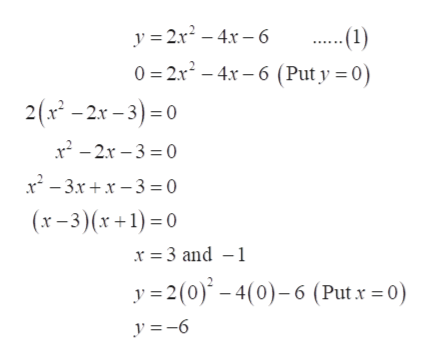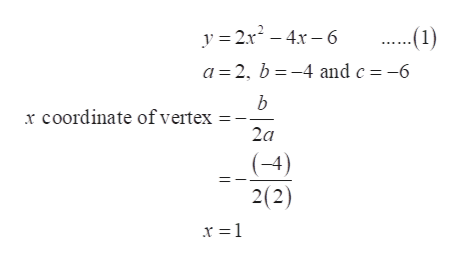# For the function y=2x2-4x-6, determine the coordinates of the x- intercept and the y- intercept.b) determine the equation of the axis of symmetryc) determine the coordinate of the vertexe) graph the function and label and indicate an appropriate scale on the axes

Question
16 views

For the function y=2x2-4x-6, determine the coordinates of the x- intercept and the y- intercept.

b) determine the equation of the axis of symmetry

c) determine the coordinate of the vertex

e) graph the function and label and indicate an appropriate scale on the axes

check_circle

Step 1

First of all, to find the x-intercept put y = 0 in equation (1) and find x.

Similarly, to find the parabola’s y-intercept put x = 0 in equation (1) and simplify to get y.

Therefore, x-intercept is (-1, 0) and (3, 0) and y-intercept is (0, -6).help_outlineImage Transcriptionclosey 2r246 -(1) 0 2x2-4x-6 (Put y 0) 2(x2-2x-3)0 x22x-30 x2-3x+x3=0 (x-3)(x+1)0 x 3 and 1 y 2(0)-4(0)-6 (Putx 0) y =6 fullscreen
Step 2

(b) Now, the symmetry axis is defined as the vertical line or horizontal line which passes through the parabola’s vertex.

Therefore, the symmetry axis is at x = 1.help_outlineImage Transcriptionclosey 2x2-4x6 -1) a 2, b-4 and c -6 b x coordinate of vertex 2a (-4) 2(2) x = 1 fullscreen
Step 3

(c) The vertex’s x-coordinate is at x = 1. So put the value o...

### Want to see the full answer?

See Solution

#### Want to see this answer and more?

Solutions are written by subject experts who are available 24/7. Questions are typically answered within 1 hour.*

See Solution
*Response times may vary by subject and question.
Tagged in

### Other